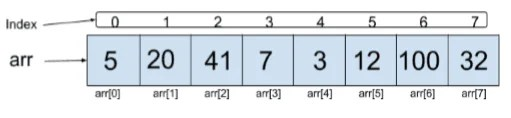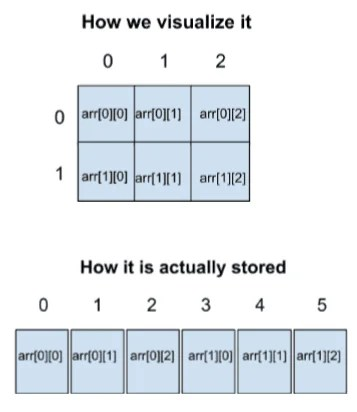View Course Path

# Arrays in C – Full explanation with examples and tutorials

Ever wanted to store a lot of numbers but thought using variables was a  lot of work? Great! It is time for you to learn Arrays in C. It is the most commonly used data structure in every programming language. In this article, we will cover arrays explanation with their types, implementation and a lot of examples.

Contents

## What are arrays?

Arrays are containers used for storing data in them. For example, we can have an array of integers or we can have an array of characters, etc. Can we have an array containing a mix of both integers and characters? No.  An array always contains the same type of data, i.e. elements of an array must all have the same data type.

## Why and how do we use arrays in C?

Let’s say the user wants to input 100 numbers. Now, how are we going to receive these inputs? In variables?

If we use variables, we will have to write this line ​

`scan("%d", &num); ​`

a  hundred times, each with a different variable name. Managing these hundred variables is a lot of hard work! Using arrays, it becomes a lot easier, let’s see how.

Just like a variable, an array needs to be declared. To declare an array, we need to specify it’s data type and size. Examples:

An array of integers of size 100:

`​int arr [ 100 ] ;`

Here’s an array of float of size 20:

`​float myArray [ 20 ] ;`

Finally, this is an array of characters of size 700:  ​

`char str [ 700 ] ;`

Note that the size of the arrays should be a constant and not a variable.  The size should be known to the compiler at the compile time.  So writing this is a big no-no :

```int n = 10 ;

int arr [ n ] ;```

After we have declared the array, we can use them in our program. There are certain operations or ways in which we can use or access elements of an array. Let’s take a look.

### Storage of  elements in the array

So to be able to use arrays, let’s first understand them through a diagram.  This is an array of integers of size 8. Yes! The size is 8. The indexing in arrays starts from 0 and not from 1. So the elements of an array lie from an index of 0 to (size – 1).Each element in an array is represented by ​arr[index]​. So in the above array, arr is 5, arr is 20, arr is 41 and so on.

To use arrays, we can do the following:

```arr = 234;

printf("%d ", arr);

int v1 = 8 * arr;```

Now, consider the same problem that we talked about. How will we receive  100 integer inputs from the user? Here is how we can code it using arrays:

```int A ;
for(int i=0; i<100; i++)
{
scanf("%d", &A[i]);
}```

Easy peasy, isn’t it?

Note that if we access elements in an array with index out of bounds, then the compiler will not throw an error. For instance, if we make an array of size 10 and try to access the 11th element, then the compiler will not throw any error. However, this is a bad practice. We should always make sure that we are not accessing a memory that does not belong to us. It is always a good practice in programming to access memory that belongs to us, otherwise, the program can end unexpectedly or even crash.

## How are arrays implemented in C?

Now let’s see how are arrays actually stored in memory. Arrays are stored as a contiguous block of memory, and the address of this contiguous block is present in the ​arr​ variable. This address is called the base address or the starting address. If you know what pointers are, then you would think that arr is a pointer variable as it contains the address of this contiguous block of memory! But, NO mate, arrays, and pointers are two completely different things.

So when we write ​arr[index]​, it is referring to the element in this contiguous memory block which is ‘index’ elements away from the starting address.  That is why indexing in an array starts from zero because the index is used as an offset. For instance, let’s say the base address is 1000. This means the address of ​arr ​ is 1000. Since the size of an integer is 4 bytes and ​arr ​ is an integer array, the address of arr will be 1004, arr will be 1008, arr  will be 1012 and so on.

To calculate the address of a[i] element, the formula used is :

`Address of a[i] = base address + (i x size of data type)`

Example: If the base address is 20 and the size of data type is 8, then:

Address of a = 20 + ( 3 x 8 ) = 44

## Types of arrays

Arrays can be of 2 types:

• one-dimensional arrays and
• multi-dimensional  arrays

One-dimensional arrays are what we have studied till now. These are used to store & access data in linear form.

Multi-dimensional arrays are also called Matrix, they can have multiple subscripts. For example a 2D array, matrix or arr. These arrays are stored in a linear manner in the memory but are visualized as matrices so as to understand the concept.

Let’s take an example,

int arr;To calculate the address of a[i][j] element, the formula used is :

`Address of a[i][j] = base address + (i x column + j) x size of data type`

Example: If the base address is 400, column = 4, size of data type = 4, then:

Address of A = 400 + ( 2 x 4 + 3 ) x 4 = 444

## Write a program to calculate the sum of 50 numbers entered by the user

```#include<stdio.h>
#include<conio.h>
void main()
{
clrscr();
int sum = 0;
for(int i=0;i<50;i++)
{
sum += arr[i];
}
printf("%d", sum);
getch();
}```

## Program to compute the average of first 200 numbers

```#include<stdio.h>
#include<conio.h>
void main()
{
clrscr();
float avg= 0.0;
for(inti=0; i<200; i++)
{
avg += arr[i];
}
avg = avg/200;
printf("%d", avg);
getch();
}```

## Write a program to find the maximum element in an array

```#include<stdio.h>
#include<conio.h>
void main()
{
clrscr();
int maxel = arr;
for (int i=1; i<100; i++)
{
if (arr[i]>maxel)
{
maxel=arr[i];
}
}
printf("%d", maxel);
getch();```

## Program to store 10 numbers in an array

```//take input of 10 numbers in array and print them
#include<stdio.h>
#include<conio.h>
void main()
{
clrscr();
int i, a;
printf("Enter the 10 number of the array\n");
for(i=0;i<10;i++)
{
scanf("%d", &a[i]);
}
printf("The elements of the Array are\n");
for(i=0;i<10;i++)
{
printf("A[%d]=%d\n",i,a[i]);
}
getch();
}
```

## Next, write a program to store 10 numbers in an array and print them in the reverse order

```//take the input of 10 numbers from user and print in reverse order
#include<stdio.h>
#include<conio.h>
void main()
{
clrscr();
int a,i;
printf("enter the 10 numbers to be printed in reverse\n");
for(i=0;i<10;i++)
{
scanf("%d",&a[i]);
}
printf("The reverse order of the numbers are\n");
for(i=9;i>=0;i--)
{
printf("%d\n",a[i]);
}
getch();
}
```

## Write a program to store numbers in two arrays. Add the contents of the two arrays and store the result in a third array.

```//take data in two arrays from user, add them, and store result in third array
#include<stdio.h>
#include<conio.h>
void main()
{
clrscr();
int a,b,sum,i;
printf("Enter elements of first array\n");
for(i=0;i<5;i++)
{
scanf("%d",&a[i]);
}
printf("Enter elements of second array\n");
for(i=0;i<5;i++)
{
scanf("%d",&b[i]);
}
for(i=0;i<5;i++)
{
sum[i]=a[i]+b[i];
}
printf("The sum of the two arrays is\n");
for(i=0;i<5;i++)
{
printf("%d\n",sum[i]);
}
getch();
}
```

## Write a program in C to store find the squares of the elements in an array

```//take 10 numbers in an array and print square of the numbers in the same array
#include<stdio.h>
#include<conio.h>
#include<math.h>
void main()
{
clrscr();
int a,i;
printf("Enter the elements of the array which you want squared\n");
for(i=0;i<10;i++)
{
scanf("%d",&a[i]);
}
printf("The squared array is \n");
for(i=0;i<10;i++)
{
a[i]=pow(a[i],2);
printf("%d\n",a[i]);
}
getch();
}
```

## How can you find if the numbers in an array are positive, negative, even or odd?

```//take 10 numbers in an array and print how many positive,negative,even and odd
#include<stdio.h>
#include<conio.h>
void main()
{
clrscr();
int a,i,countpos=0,countneg=0,counteven=0,countodd=0,countevenodd=0;
printf("Enter 10 varied integers for an array\n");
for(i=0;i<10;i++)
{
scanf("%d",&a[i]);
if(a[i]>=0)
{
countpos++;
}
else
{
countneg++;
}
countevenodd=a[i]%2;
if(countevenodd==0)
{
counteven++;
}
else
{
countodd++;
}
}
printf("Even numbers = %d\n",counteven);
printf("Odd numbers = %d\n",countodd);
printf("Positive numbers = %d\n",countpos);
printf("Negative numbers = %d\n",countneg);
getch();
}
```

## Write a program in C to find the largest and smallest element in an array

```//Take an array from the user and find the largest and smallest
#include<stdio.h>
#include<conio.h>
void main()
{
clrscr();
int i,greatest,lowest,a;
printf("Enter the elements of an array\n");
for(i=0;i<10;i++)
{
scanf("%d",&a[i]);
}
greatest = a;
for(i=1;i<10;i++)
{
if(greatest>a[i])
greatest = greatest;
else
greatest = a[i];
}
printf("Greatest=%d",greatest);
lowest = a;
for(i=1;i<10;i++)
{
if(lowest<a[i])
lowest=lowest;
else
lowest=a[i];
}
printf("\nLowest=%d",lowest);
getch();
}
```

## Write a program using C to arrange the elements of an array in an ascending order

```//take in 10 elements of an array and rearrange the array in increasing order
#include<stdio.h>
#include<conio.h>
void main()
{
clrscr();
int i,a,temp;
printf("Enter the elements of the array\n");
for(i=0;i<10;i++)
{
scanf("%d", &a[i]);
}
for(int j=0;j<10;j++)
{
for(i=0;i<10;i++)
{
if(a[i]<a[i+1])
{
a[i]=a[i];
}
else
{
temp=a[i];
a[i]=a[i+1];
a[i+1]=temp;
}
}
}
printf("The array arranged in increasing order is\n");
for(i=0;i<10;i++)
{
printf("%d\n",a[i]);
}
getch();
}```

This site uses Akismet to reduce spam. Learn how your comment data is processed.